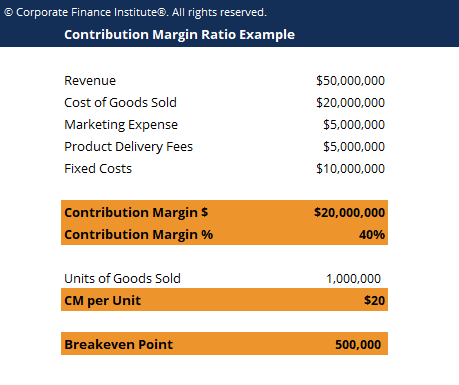# Contribution Margin Ratio Template

## Contribution Margin Ratio Template

This contribution margin ratio template shows you how to calculate the contribution margin, CM percentage and breakeven point.

Here is the screenshot of the contribution margin ratio:### Contribution Margin Ratio Template

The contribution margin ratio (CM ratio) of a business is equal to its revenue less all variable costs divided by its revenue. It represents the marginal benefit of producing one more unit.

The formula for contribution margin ratio is:

CM ratio = (total revenue – cost of goods sold – any other variable expenses) / total revenue

### Breakeven analysis

Contribution margin is not necessarily a good indication of economic benefit.  Companies may have significant fixed costs that need to be factored in. It can be important to perform a break even analysis to determine how many units need to be sold and at what to breakeven.

In order to perform this analysis, calculate the contribution margin per unit, then divide the fixed costs by this number and you will know how many units you have to sell to break even.

### More Free Templates

For more resources, check out our business templates library to download numerous free Excel modeling, PowerPoint presentation and Word document templates.

• Excel Modeling Templates
• PowerPoint Presentation Templates
• Transaction Document Templates

### Financial Analyst Certification

Become a certified Financial Modeling and Valuation Analyst (FMVA)® by completing CFI’s online financial modeling classes and training program!